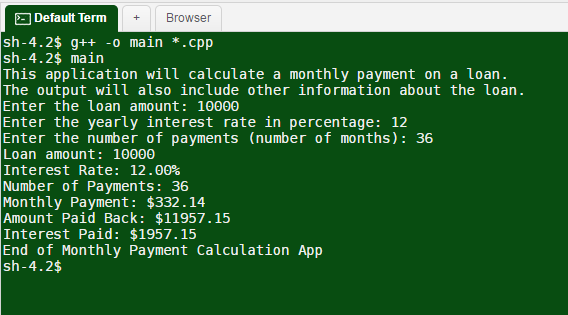# Answered! Write a program named, paymentCalculator.cpp. This program will first print a description of what the…

Write a program named, paymentCalculator.cpp. This program will first print a description of what the program does. Next, this program will prompt for and input from the user the amount of the loan, the yearly interest on the loan (in percent, like 6% or 8%, not .06 or .08 – the program will have to make that adjustment as it executes), and the number of months (or payments) in the term of the loan. Now the program will calculate the amount of one monthly payment using the formula:Don't use plagiarized sources. Get Your Custom Essay on
Answered! Write a program named, paymentCalculator.cpp. This program will first print a description of what the…
GET AN ESSAY WRITTEN FOR YOU FROM AS LOW AS \$13/PAGE

– where rate is the monthly interest rate
(yearly interest rate divided by 12), n is the number of months or
payments.

The monthly payment is the variable, payment, and the amount of the loan
is principle.

Once the monthly payment is calculated, the program will display the following information, formatted as in the example below: the loan amount, the yearly interest rate, the number of payments, the amount of the monthly payment, the amount of money that will be paid back once all payments have been made, and the total amount of interest paid once the loan has been fully repaid.

Here are three examples. The output of your program should be formatted like these examples and work for any inputs received from the user. This program will assume the user’s inputs are valid.

Example 1:

```This application will calculate a monthly payment on a loan.
The output will also include other information about the loan.
Enter the loan amount: 10000
Enter the yearly interest rate in percents: 12
Enter the number of payments (number of months): 36
Loan Amount:         \$  10000.00
Interest Rate:             12.00%
Number of Payments:           36
Monthly Payment:     \$    332.14
Amount Paid Back:    \$  11957.15
Interest Paid:       \$   1957.15
End of Monthly Payment Calculation App
```

#include<iostream>

#include<iomanip>
#include<math.h>

using namespace std;

int main()
{
cout << “This application will calculate a monthly payment on a loan.” << endl;
cout << “The output will also include other information about the loan.” << endl;

double YearlyInt, LoanAmount, Payment, AmountPaid;

int NumPayments;

cout << “Enter the loan amount: “;
cin >> LoanAmount;

cout << “Enter the yearly interest rate in percentage: “;
cin >> YearlyInt;

cout << “Enter the number of payments (number of months): “;
cin >> NumPayments;

cout << “Loan amount: ” << LoanAmount << endl;
cout << “Interest Rate: ” << fixed << setprecision(2) << YearlyInt << “%” << endl;
cout << “Number of Payments: ” << NumPayments << endl;

// per month payment
Payment = LoanAmount * YearlyInt/(12*100) * pow( 1 + YearlyInt/(12*100), NumPayments);
Payment /= pow( 1 + YearlyInt/(12*100), NumPayments) – 1;

// total payment
AmountPaid = Payment * NumPayments;
cout << “Monthly Payment: \$” << Payment << endl;
cout << “Amount Paid Back: \$” << AmountPaid << endl;
cout << “Interest Paid: \$” << (AmountPaid – LoanAmount) << endl;

cout << “End of Monthly Payment Calculation App ” << endl;

return 0;
}